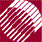ECE532 Biomedical Optics © 1998 Steven L. Jacques, Scott A. Prahl Oregon Graduate Institute

# Angular patterns of Mie scattering

Consider the scattering pattern from a 0.304-µm-dia. nonabsorbing polystyrene sphere in water irradiated by HeNe laser beam:
 np = 1.5721 particle refractive index nmed = 1.3316 medium refractive index a = 0.152 µm, particle radius= 0.6328 µm wavelength in vacuo

As calculated before:
 nr = np/nmed = 1.5721/1.3316 = 1.1806 relative refractive index x = 2πa/(λ>/nmed) = (2)(3.1415)(0.152)/(0.6328/1.3316) = 2.0097 size parameter

### Run the Mie theory algorithm:

Mie(nr, x) ---> S1(θ), S1(θ) as complex numbers

Mie(1.1806, 2.0097) ---> S1.re + jS1.im, S2.re + jS2.im as functions of θ

To view the results, calculate the intensities of scattering for parallel and perpendicular orientations of polarized source/detector pairs:

Ipar = S2S2* = Re{(S2.re + jS2.im)(S2.re - jS2.im)}

Iper = S1S1* = Re{(S1.re + jS1.im)(S1.re - jS1.im)}

which are shown in the following figures, and can be experimentally measured as described below.

The following figures describe the experimental measurements that illustrate the angular dependence of the scattered intensities Ipar and Iper:

## Iper

Irradiate dilute solution of spheres in water with laser beam polarization oriented perpendicular to the table. Collect scattered light as a function of angle θ in plane parallel to table. Place linear polarization filter in front of detector perpendicular to the table.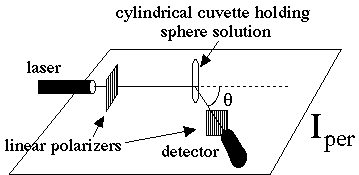## Ipar

Irradiate dilute solution of spheres in water with laser beam polarization oriented parallel to the table. Collect scattered light as a function of angle θ in plane parallel to table. Place linear polarization filter in front of detector parallel to the table.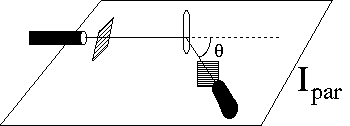### Example results: Polar and xy plots of scattering pattern for Ipar and Iper

Polar plot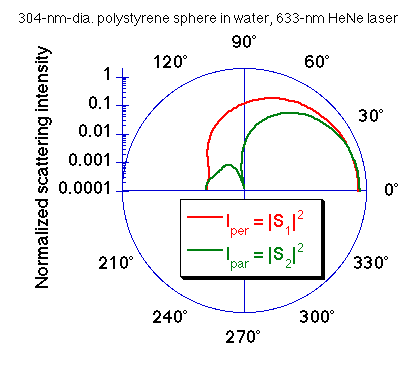I(θ) plot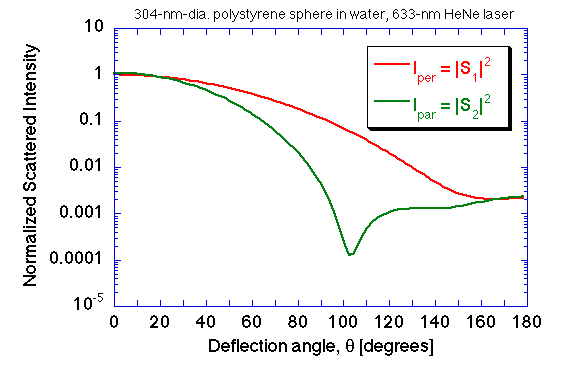For a randomly polarized light source, the total scattered light intensity is given by the term S11: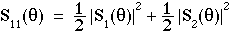S11 is the first element of the so-called Mueller Matrix, a 4x4 matrix which relates an input vector of Stokes parameters (Ii, Qi, Ui, Vi) describing a complex light source and the output vector (Is, Qs, Us, Vs) describing the nature of the transmitted light. For randomly polarized light, S11 describes the transport of total intensity:

Is = S11Ii